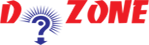﻿ What is a Command: A Brief Intro with an Example## Operators - Introduction

The common binary operators for arithmetic are + for addition, - for subtraction, * for multiplication, and / for division. As already mentioned, Python uses ** for exponentiation. Integer division is performed so that the result is always another integer (the integer quotient):

``````
>>> 25/3
8
>>> 5/2
2
```
```

Always remember, to get accurate answer when working with Python, use the float type:

``````
>>> 25.0/3
8.3333333333333339
>>> 5/2.0
2.5
```
```

If just one of the operands is of type float, then the result will be of type float. For example:

``````
>>> 2**(1/2)
1
```
```

where we wanted to compute the square root of 2 as the 1/2 power of 2, but the division in the exponent produced a result of 0 because of integer division. A correct way to do this computation is:

``````
>>> 2**0.5
1.4142135623730951
```
```

Another useful operator is %, which is read as ”mod”. This gives the remainder of an integer division, as in

``````
>>> 5 % 2
1
>>> 25 % 3
1
```
```

which shows that 5 mod 2 = 1, and 25 mod 3 = 1. This operator is useful in number theory and cryptography.

Apart from the arithmetic operators we need comparison operators: <, >, <=, >=, ==, !=, <>. These are read as: is less than, is greater than, is less than or equal to, is greater than or equal to, is equal to, is not equal to, is not equal to.

The result of a comparison is always a boolen value that is True or False.

``````
>>> 2 < 3
True
>>> 3 <2
False
>>> 3 <= 2
False
```
```

Note: The != and <> operators are synonomous; both means not equal to. Also, the operator == means is equal to.

``````
>>> 2 <> 3
True
>>> 2 != 3
True
>>> 0 != 0
False
>>> 0 == 0
True
```
```

PythonTraining, 258-FF , Katewa Nagar, New Sanganer Rd, Jaipur, 302019